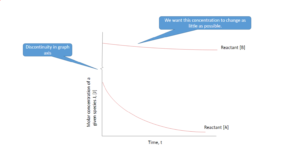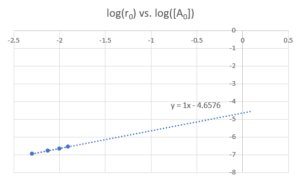# 13 Reaction Order

Learning Objectives

By the end of this section, you should be able to:

Define reaction order and overall order

Determine the reaction order and the rate constant from kinetic data

The dependence of the rate of reaction on the reactant concentrations can often be expressed as a direct proportionality, in which the concentrations may be raised to be the zeroth, first, or second power. The exponent is known as the order of the reaction with respect to that substance.$^{}$

The overall order of a reaction is the sum of the orders with respect to the sum of the exponents

For example:

$r = k_{r}[A]^3[B]^{1/2}$

The reaction is:

• Third-order in A
• One-half order in B
• Three and a half order overall

In the more complicated example for the reaction previously given between hydrogen and bromine:

$r = \frac{k_{a}[H_{2}][Br_{2}]^{3/2}}{[Br_{2}]+k_{b}[H\!Br]}$

The reaction is:

• First order in $H_{2}$
• Indeterminate order in $Br_{2}$(as it’s not in a single term)
• Indeterminate order in $H\!Br$ (as it cannot be isolated to a single term raised to a power)
• Indeterminate order overall (as some orders are indeterminate)

## Determining the Rate Law

### Isolation Method for Determining the Rate Law

One of the simplest methods for determining the rate law is the isolation method.

Say we have a reaction with two reactants, A and B. We put a large amount of B in our reactor compared to A; so much that the concentration barely changes.

If the true rate law is: $r = k_{r}[A][B]^2$

What we would observe is $r = k_{eff}[A]$, where $k_{eff}=k_{r}[B_{0}]^2$

• $k_{eff}$ = the effective rate constant
• $[B]_{0}$indicating the concentration of B at the start of the reaction (or time zero) or also called B naught (with naught meaning nothing or zero in this case)With B in great excess: $r = k_{eff}[A]$ where $k_{eff}=k_{r}[B_{0}]^2$, reaction appears first order.

With A in great excess: $r' = k'_{eff}[B]^2$ where $k'_{eff}=k_{r}[A_{0}]$, reaction appears second order.

$k'_{eff}$ is read as k prime effective

It is easier to analyse these individual effective rate laws than the more complex combined rate law when both concentrations change significantly.

### Method of Initial Rates

The method of initial rates is commonly used in conjunction with the isolation methods to determine reaction order.

Exercise: Method of Initial Rates

Say we have a reaction with two species, A and B. We put in the same amount of B into the reactor each time. The following is our initial rates are observed with different concentrations of A. What is the reaction order in A?

 [A] (mol/L) Initial rate(mol/L·s) 1 $1×10^{-2}$ 2 $4×10^{-2}$

### Solution

As the concentration of A doubles, the reaction rate quadruples

$r_{0}=k_{eff}[A]^2$

So the reaction is second order in A.

If we take the logarithm of the general equation for reaction rate, we can linearize the equation:

\begin{align*}
r_{0} & = k_{eff}[A_{0}]^a \\
log(r_{0})& = log(k_{eff}) + a*log([A_{0}])\\
y & = intercept + slope * x
\end{align*}

Plot $log(r_{0})$ vs $log([A_{0}])$, where the slope is the reaction order in A; and y-intercept equals to $log(k_{eff})$

Exercise: Reaction Order and Rate Constant

Sulfuryl chloride ($SO_{2}Cl_{2}$) decomposes to $SO_{2}$ and $Cl_{2}$ by the following reaction:

$SO_{2}Cl_{2(g)} → SO_{2(g)}+Cl_{2(g)}$

Data for the reaction at 320°C are listed in the following table. Calculate the reaction order with regard to sulfuryl chloride and determine the rate constant for the reaction.$^{}$

 Experiment $[SO_{2}Cl_{2}]_{0}$ Initial rate(M/s) 1 0.0050 $1.10×10^{-7}$ 2 0.0075 $1.65×10^{-7}$ 3 0.0100 $2.20×10^{-7}$ 4 0.0125 $2.75×10^{-7}$

### Solution

Step 1: determine the reaction order with respect to sulfuryl chloride

Comparing Experiments 1 and 3, for example, shows that doubling the concentration doubles the reaction rate $(2.20×10^{-7}) ÷ (1.10×10^{-7}) = 2.0$, which means that the reaction rate is proportional to $[SO_{2}Cl_{2}]$.

The reaction is first order with respect to  $[SO_{2}Cl_{2}]$.

Step 2: calculate $k_{r}$

We have $rate = k_{r}[SO_{2}Cl_{2}]$. We can calculate the rate constant ($k_{r}$) using data from any experiment in the table.
\begin{align*}
rate & = k_{r}[SO_{2}Cl_{2}] \\
1.10×10^{-7} M/s& = k_{r}×0.0050M \\
2.2×10^{-5}s^{-1} & = k_{r}
\end{align*}

Method 2

The following graph is produced when plotting $log(r_{0})$ vs $log([A_{0}])$From the line of best fit, we can see the slope is 1 (meaning a first order relationship) and $log(k_{r})=-4.6576$

Therefore the reaction is first order in $SO_{2}Cl_{2}$ , $k_{r}=10^{-4.6576} = 2.2×10^{-5}s^{-1}$.

## References